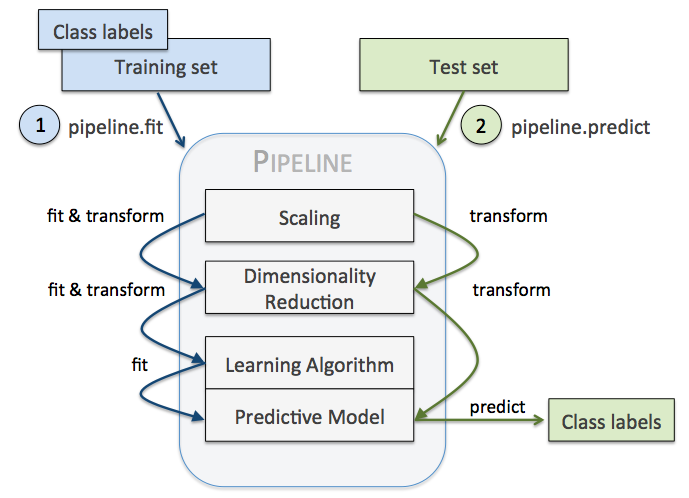• 1、源数据ETL
• 2、数据预处理
• 3、特征选取
• 4、模型训练与验证

## 1. 加载数据集

``````from pandas as pd
from sklearn.cross_validation import train_test_split
from sklearn.preprocessing import LabelEncoder

# Breast Cancer Wisconsin dataset

X, y = df.values[:, 2:], df.values[:, 1]
# y为字符型标签
# 使用LabelEncoder类将其转换为0开始的数值型
encoder = LabelEncoder()
y = encoder.fit_transform(y)
>>> encoder.transform(['M', 'B'])
array([1, 0])
X_train, X_test, y_train, y_test = train_test_split(X, y, test_size=.2, random_state=0)``````

## 2. 构思算法的流程

• 特征标准化是需要的，可作为第一个环节
• 既然是分类器，classifier也是少不了的，自然是最后一个环节
• 中间可加上比如数据降维（PCA）
``````from sklearn.preprocessing import StandardScaler
from sklearn.decomposition import PCA
from sklearn.linear_model import LogisticRegression

from sklearn.pipeline import Pipeline

pipe_lr = Pipeline([('sc', StandardScaler()),
('pca', PCA(n_components=2)),
('clf', LogisticRegression(random_state=1))
])
pipe_lr.fit(X_train, y_train)
print('Test accuracy: %.3f' % pipe_lr.score(X_test, y_test))

# Test accuracy: 0.947``````

Pipeline对象接受二元tuple构成的list，每一个二元 tuple 中的第一个元素为 arbitrary identifier string，我们用以获取（access）Pipeline object 中的 individual elements，二元 tuple 中的第二个元素是 scikit-learn与之相适配的transformer 或者 estimator。

``Pipeline([('sc', StandardScaler()), ('pca', PCA(n_components=2)), ('clf', LogisticRegression(random_state=1))])``

## 3. Pipeline执行流程的分析

Pipeline 的中间过程由scikit-learn相适配的转换器（transformer）构成，最后一步是一个estimator。比如上述的代码，StandardScaler和PCA transformer 构成intermediate steps，LogisticRegression 作为最终的estimatorSklearn中的pipeline